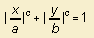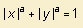# backgrounds

## main

history
I collected curves when I was a young boy. Then, the papers rested in a box for decades. But when I found them, I picked the collection up again, some years spending much work on it, some years less.

questions
I have been thinking a long time about two questions:

1. what is the unity of curve?
Stated differently as: when is a curve different from another one?
2. which equation belongs to a curve?

1. unity of curve
I decided to aim for simplicity: it does not matter when a curve has been reformatted  in a linear way (by ways of translation, rotation or multiplication).
This means that I omit constants in the equations of a curve, as been found by other authors.

Example:
for me the equation of the super ellipse is notbut:Only the parameter 'a' affects the form of the curve.
And all linear transformations of this curve do belong to this same curve 'family'.

2. which formula
I don't want to swim in an ocean of formulae.
Therefore I look for a formula that is as simple as possible, for covering a given curve. Trying to confine myself to Cartesian, polar, bipolar and parametric equations.
Examples:

Sometimes the definition of a curve can not fit in one of these forms:

• textual definition: let there be etc.
Example: apply the following rule to a grid of black squares: when you get on a black square, make it white and turn to the right; when you get on a white square, make it black and turn to the left (ant of Langton)

Sometimes a much shorter or much more elegant formula can be found, using another way of defining a curve:

literature
I made up a list of the literature I used.